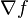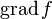In vector calculus, the gradient of a multivariate function measures how steep a curve is. On a graph of the function, it is the slope of the tangent of that curve. More generally, it is a vector that points in the direction in which the function grows the fastest. Its coordinates are partial derivatives of that function. The gradient of a function f is often written as$\nabla f$ or$\operatorname{grad} f$.